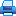Address: office 1410a, 17 Naberezhnaya Severnoy Dviny, Arkhangelsk, 163002, Russian Federation, Northern (Arctic) Federal University named after M.V. Lomonosov

Phone: (818-2) 21-61-21
E-mail: vestnik_est@narfu.ru
http://aer.narfu.ru/en/

# The Quadrangle Midlines, or Attractive Useless Synthesis. P. 109–117Section: Physics. Mathematics. Informatics

514.112.4

### Authors

Yastrebov Aleksandr Vasil'evich
Yaroslavl State Pedagogical University named after K.D. Ushinsky (Yaroslavl, Russia)
е-mail: a.yastrebov@yspu.org
Shabanova Mariya Valer'evna
Institute of Mathematics, Information and Space Technologies, Northern (Arctic) Federal University named after M.V. Lomonosov (Arkhangelsk, Russia)
е-mail: m.shabanova@narfu.ru

### Abstract

The paper deals with new results concerning the geometry of a convex quadrangle. Considering mathematics as a body of knowledge, we introduce the concept of “the midline of a convex quadrangle” and “the n-th order middle of a convex quadrangle”. The formula expressing the area of a convex quadrangle through the lengths of its midlines and some other numerical characteristics is proved. The peculiarity of the resulting formula is a combination of 12 numerical characteristics. Several hypotheses concerning the sequence of midpoints of a convex quadrangle are formulated and experimentallyconfirmed. Thus, we indicate a possible study direction of the geometry of a quadrangle. Considering mathematics as an area of human activity, the paper presents the logic of statement and solution of the investigative problem in the selected area of geometry. In the opinion of the authors the mathematiciantheorist and mathematician-experimentalist activities are of great interest. The paper deals with the experimental roots of mathematics. Methodology of the paper includes a complex of complementary methods: considerations of the alteration of figures, comparison of some mathematical formulas from the viewpoint of their stability under deformations, a method of theoretical synthesis, and experimental methods. All hypotheses for the convex quadrangle properties have been obtained as a result of the computer experiment in the interactive geometric environment "GeoGebra". Hence, the use of computers generates new investigative ideas in the elementary geometry.

### Keywords

elementary geometry, convex quadrangle, synthesis, computer experiment, multitemporal model